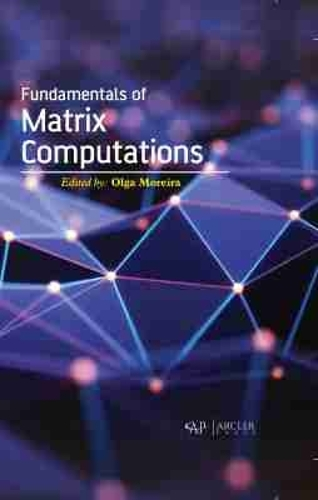•# Fundamentals of Matrix Computations (Hardback)

(editor)
£160.00
Hardback 290 Pages / Published: 30/11/2019
• We can order this

Usually dispatched within 2 weeks

Fundamentals of Matrix Computations deals with the concept of matrix computations, a technique of singular value homogenization and its application in medical therapy. It consists of modern iterative methods to generalize the issues associated with singular-value homogenization. It provides the reader with the understanding of matrix computations and preconditioning technique of singular value homogenization so as to analyze its potential applications in the field of medical therapy and the use of efficient numerical methods so as to solve the problems linked with nonlinear singular boundary value by using improved differential transform method. This book also discusses about blind distributed estimation algorithms for adaptive networks, a dft-based approximate eigenvalue and singular value decomposition of polynomial matrices, sparse signal subspace decomposition based on adaptive over-complete dictionary, lower bounds for the low-rank matrix approximation and a semi-smoothing augmented lagrange multiplier algorithm for low-rank toeplitz matrix completion.

Publisher: Arcler Education Inc
ISBN: 9781774071441
Number of pages: 290
Dimensions: 229 x 152 mm